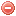# Description

The 1-hour timelag fuel moisture $$MC_1$$ is the moisture content of the 1-hour timelag fuels, which consist of fine dead fuels, i.e. dead herbaceous plants, roundwood less than 0.25 inch in diameter, and the uppermost layer of litter on the forest floor (Deeming et al. 1977).

The calculation of the 1-hour timelag fuel moisture model requires the use of fuel sticks. However, an estimation of the 1-hour fuel moisture at midafternoon without the use of fuel sticks is possible according to the formula described here (Bradshaw et al. 1983).

The calculation of the 1-hour timelag fuel moisture model requires daily temperature [°F], relative humidity [%] and fraction of sky cover at early to midafternoon time (Bradshaw et al. 1983).

# Formula

This type of fuels responds so quickly to changing environmental conditions that only the equilibrium moisture content $$EMC_{f/a}$$ at the fuel-atmosphere interface is required for calculating the 1-hour timelag fuel moisture (Cohen & Deeming 1985).

The equilibrium moisture content [%] at the fuel-atmosphere interface $$EMC_{f/a}$$ is a function of air temperature $$T_{f/a}$$ [°F] and relative humidity $$H_{f/a}$$ [%] in immediate contact with the fuel elements. $$T_{f/a}$$ and $$H_{f/a}$$ are estimated by correcting air temperature $$T$$ and relative humidity $$H$$ at instrument heigt (4.5 ft) according to cloud cover. The temperature correction has to be added to $$T$$ in order to obtain $$T_{f/a}$$, and the relative humidity correction has to be multiplied by $$H$$ in order to obtain $$H_{f/a}$$ according to the following adjustment factors (Bradshaw et al. 1983):

Variable Fraction of sky cover
0.0-0.1 0.1-0.5 0.6-0.9 0.9-1.0
Temperature [°F] + 25 + 19 + 12 + 5
Relative humdity [%] · 0.75 · 0.83 · 0.91 · 1.00

Then, $$EMC_{f/a}$$ is obtained by substituting $$T$$ and $$H$$ by $$T_{f/a}$$ and $$H_{f/a}$$ in the standard $$EMC$$ equation (cf. Equilibrium moisture content).

Finally, the 1-hour timelag fuel moisture content $$MC_1$$ [%] for midafternoon observation time is calculated as follows:

$MC_1=1.03\cdot{EMC_{f/a}}$

The 1-hour timelag fuel moisture model can be calculated also on a instantaneous basis, according to the available data.

# References:

Original publication:
Deeming et al. (1977)

Other publications:
Cohen & Deeming (1985)

### Bibliography SearchNo results!

No results were found. Are you sure you searched for a tag?

### Symbols

 Variable Description Unit $$T$$ air temperature °C $$T_{dew}$$ dew point temperature °C $$H$$ air humidity % $$P$$ rainfall mm $$U$$ windspeed m/s $$w$$ days since last rain (or rain above threshold) d $$rr$$ days with consecutive rain d $$\Delta t$$ time increment d $$\Delta{e}$$ vapor pressure deficit kPa $$e_s$$ saturation vapor pressure kPa $$e_a$$ actual vapor pressure kPa $$p_{atm}$$ atmospheric pressure kPa $$PET$$ potential evapotranspiration mm/d $$r$$ soil water reserve mm $$r_s$$ surface water reserve mm $$EMC$$ equilibrium moisture content % $$DF$$ drought factor - $$N$$ daylight hours hr $$D$$ weighted 24-hr average moisture condition hr $$\omega$$ sunset hour angle rad $$\delta$$ solar declination rad $$\varphi$$ latitude rad $$Cc$$ cloud cover Okta $$J$$ day of the year (1..365/366) - $$I$$ heat index - $$R_n$$ net radiation MJ⋅m-2⋅d-1 $$R_a$$ daily extraterrestrial radiation MJ⋅m-2⋅d-1 $$R_s$$ solar radiation MJ⋅m-2⋅d-1 $$R_{so}$$ clear-sky solar radiation MJ⋅m-2⋅d-1 $$R_{ns}$$ net shortwave radiation MJ⋅m-2⋅d-1 $$R_{nl}$$ net longwave radiation MJ⋅m-2⋅d-1 $$\lambda$$ latent heat of vaporization MJ/kg $$z$$ elevation m a.s.l. $$d_r$$ inverse relative distance Earth-Sun - $$\alpha$$ albedo or canopy reflection coefficient - $$\Delta$$ slope of the saturation vapor pressure curve kPa/°C $$Cc$$ cloud cover eights $$ROS$$ rate of spread m/h $$RSF$$ rate of spread factor - $$WF$$ wind factor - $$WRF$$ water reserve factor - $$FH$$ false relative humidity - $$FAF$$ fuel availability factor - $$PC$$ phenological coefficient -

 Suffix Description $$-$$ mean / daily value $$_{max}$$ maximum value $$_{min}$$ minimum value $$_{12}$$ value at 12:00 $$_{13}$$ value at 13:00 $$_{15}$$ value at 15:00 $$_{m}$$ montly value $$_{y}$$ yearly value $$_{f/a}$$ value at fuel-atmosphere interface $$_{dur}$$ duration $$_{soil}$$ value at soil level

 Constant Description $$e$$ Euler's number $$\gamma$$ psychrometric constant $$G_{SC}$$ solar constant $$\sigma$$ Stefan-Bolzmann constant